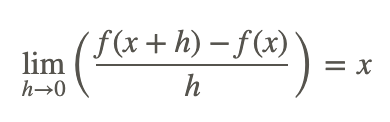# 1.1: Concept of Limit

•• Contributed by CK12
• CK12

When learning about the end behavior of a rational function you described the function as either having a horizontal asymptote at zero or another number, or going to infinity. Limit notation is a way of describing this end behavior mathematically.

You already know that as x gets extremely large then the functiongoes 8/3 because the greatest power are equal and 8/3 is the ration of the leading coefficients. How is this statement represented using limit notation?

## Introduction to Limits

Limit notation is a way of stating an idea that is a little more subtle than simply saying x=5 or y=3.The letter a can be any number or infinity. The function f(x) is any function of x. The letter b can be any number. If the function goes to infinity, then instead of writing “=∞” you should write that the limit does not exist or “DNE”. This is because infinity is not a number. If a function goes to infinity then it has no limit.

Take the following limit:

The limit of y=4x2 as x approaches 2 is 16

In limit notation, this would be:While a function may never actually reach a height of b it will get arbitrarily close to b. One way to think about the concept of a limit is to use a physical example. Stand some distance from a wall and then take a big step to get halfway to the wall. Take another step to go halfway to the wall again. If you keep taking steps that take you halfway to the wall then two things will happen. First, you will get extremely close to the wall but never actually reach the wall regardless of how many steps you take. Second, an observer who wishes to describe your situation would notice that the wall acts as a limit to how far you can go.

## Examples

### Example 1

Earlier, you were asked how to write the statement "The limit ofas x approaches infinity is 8/3" in limit notation.

This can be written using limit notation as:### Example 2

Translate the following mathematical statement into words.The limit of the sum of 1/2+1/4+1/8+⋯ as the number of terms approaches infinity is 1.

### Example 3

Use limit notation to represent the following mathematical statement.

1/3+1/9+1/27+⋯=1/2### Example 4

Describe the end behavior of the following rational function at infinity and negative infinity using limits.Since the function has equal powers of x in the numerator and in the denominator, the end behavior is −1/2 as x goes to both positive and negative infinity.### Example 5

Translate the following limit expression into words. What do you notice about the limit expression?The limit of the ratio of the difference between f of quantity x plus h and f of x and h as h approaches 0 is x.

You should notice that h→0 does not mean h=0 because if it did then you could not have a 0 in the denominator. You should also note that in the numerator, f(x+h) and f(x) are going to be super close together as h approaches zero. Calculus will enable you to deal with problems that seem to look like 0/0 and /.

## Review

Describe the end behavior of the following rational functions at infinity and negative infinity using limits.Translate the following statements into limit notation.

6. The limit of y=2x2+1 as x approaches 3 is 19.

7. The limit of y=ex as x approaches negative infinity is 0.

8. The limit of y=1/x as x approaches infinity is 0.

Use limit notation to represent the following mathematical statements.

9. 1/4+1/16+1/64+⋯=1/3

10. The series 1+1/2+1/3+1/4+⋯ diverges.

11. 1+1/2+1/4+1/8+⋯=2

12. 9/10+9/100+9/1000+⋯=1

Translate the following mathematical statements into words.## Vocabulary

Term Definition
End behavior End behavior is a description of the trend of a function as input values become very large or very small, represented as the 'ends' of a graphed function.
Horizontal Asymptote A horizontal asymptote is a horizontal line that indicates where a function flattens out as the independent variable gets very large or very small. A function may touch or pass through a horizontal asymptote.
limit notation Limit notation is a way of expressing the fact that a function gets arbitrarily close to a value.